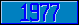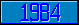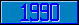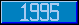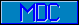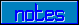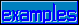## GKS - /GEVTM

### 4.23 Utility functions 4.23.1 GEVTM, Evaluate transformation matrix

control-
mnemonic
Level   GKS function name
GEVTM 1a Evaluate transformation matrix
5 parameters
p1 ::= gkspnt fixed point (WC/NDC)
p2 ::= L gksnum 2 value shift vector (WC/NDC)
p3 ::= gksnum rotation angle in radians (positive if counterclockwise)
p4 ::= L gksnum 2 value scale factor
p5 ::= gksenum coordinate switch:
 ││ WC NDC ││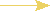Write /GevTM("1,1","1.1,0.5","1.223","2.0,1.5","WC")
1 value returned
v1 ::= L gksnum 2x3 segment transform matrix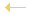Read Matrix